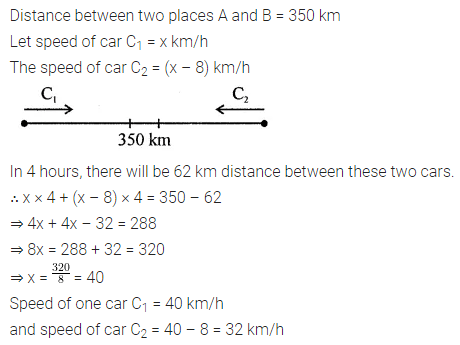# ML Aggarwal Class 8 Solutions for ICSE Maths Chapter 12 Linear Equations and Inequalities in one Variable Ex 12.2

## ML Aggarwal Class 8 Solutions for ICSE Maths Chapter 12 Linear Equations and Inequalities in One Variable Ex 12.2

Question 1.
Three more than twice a number is equal to four less than the number. Find the number.
Solution:Question 2.
When four consecutive integers are added, the sum is 46. Find the integers.
Solution: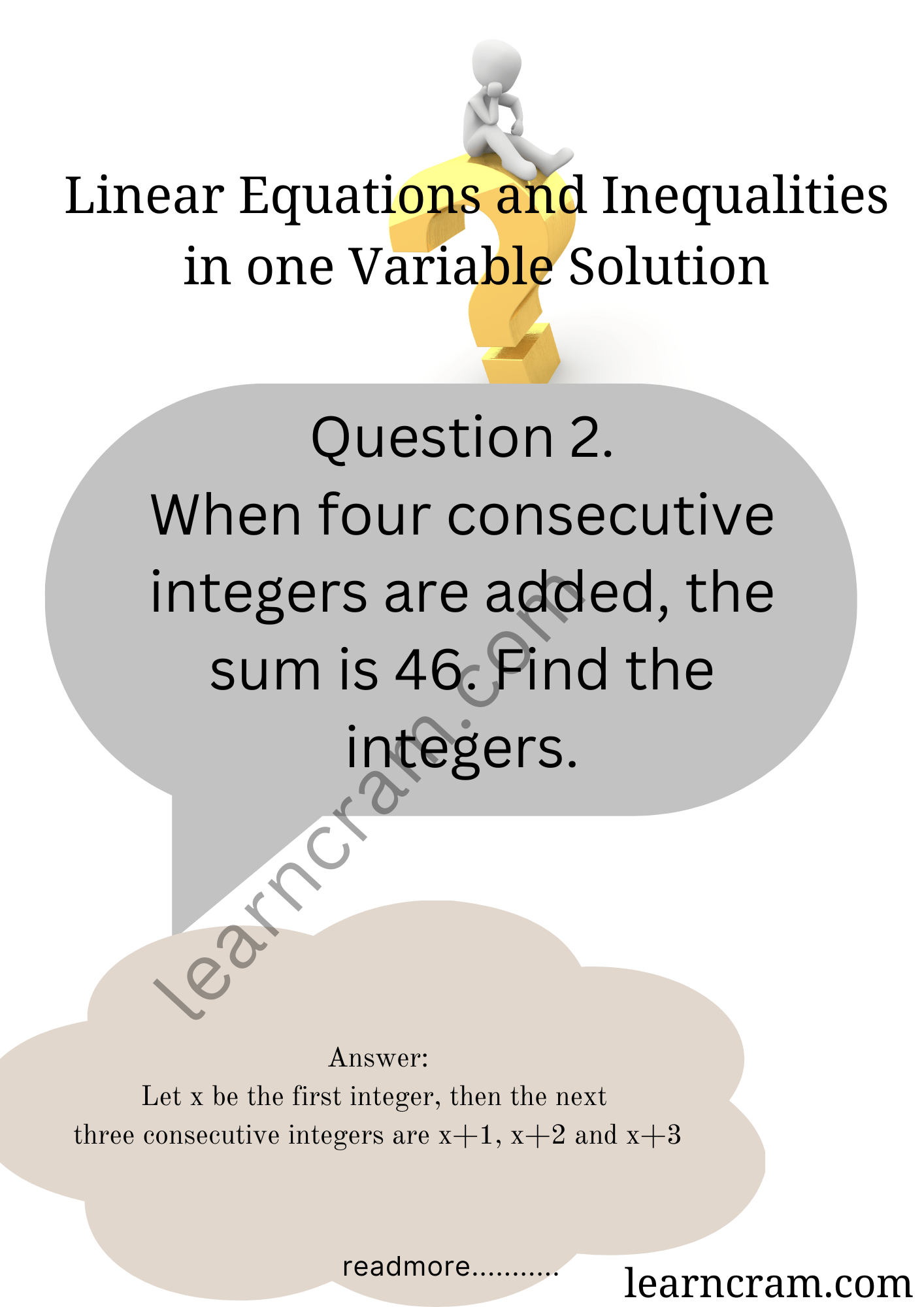Question 3.
Manjula thinks a number and subtracts $$\frac{7}{3}$$ from it. She multiplies the result by 6. The result now obtained is 2 less than twice the same number she thought of. What is the number?
Solution:Question 4.
A positive number is 7 times another number. If 15 is added to both the numbers, then one of the new numbers becomes $$\frac{5}{2}$$ times the other new number. What are the numbers?
Solution: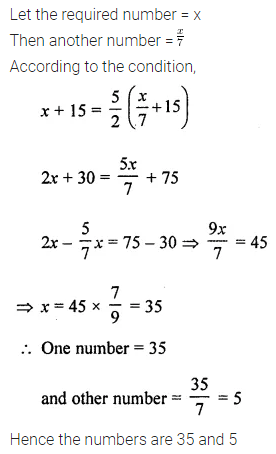Question 5.
When three consecutive even integers are added, the sum is zero. Find the integers.
Solution:Question 6.
Find two consecutive odd integers such that two-fifth of the smaller exceeds two-ninth of the greater by 4.
Solution: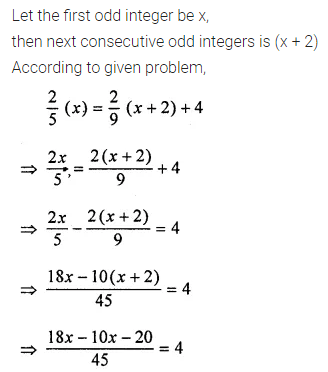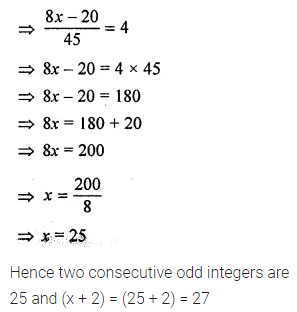Question 7.
The denominator of a fraction is 1 more than twice its numerator. If the numerator and denominator are both increased by 5, it becomes $$\frac{3}{5}$$. Find the original fraction.
Solution: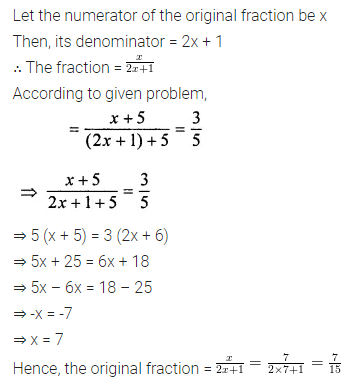Question 8.
Find two positive numbers in the ratio 2 : 5 such that their difference is 15.
Solution: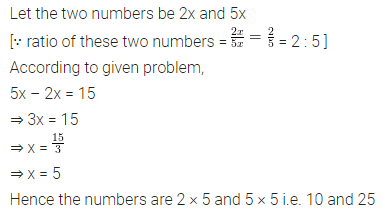Question 9.
What number should be added to each of the numbers 12, 22, 42 and 72 so that the resulting numbers may be in proportion?
Solution: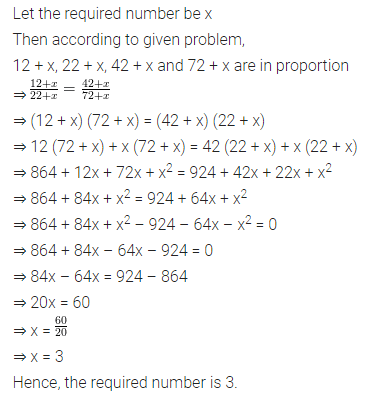Question 10.
The digits of a two-digit number differ by 3. If the digits are interchanged and the resulting number is added to the original number, we get 143. What can be the original number?
Solution: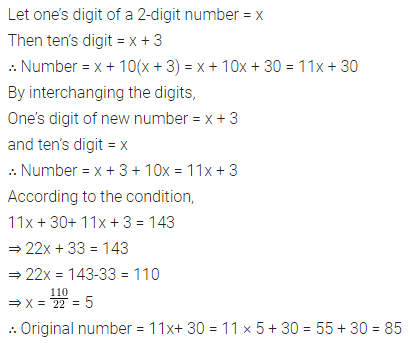Question 11.
Sum of the digits of a two-digit number is 11. When we interchange the digits, it is found that the resulting new number is greater than the original number by 63. Find the two-digit number.
Solution: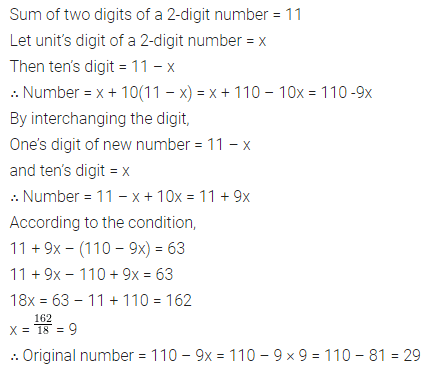Question 12.
Ritu is now four times as old as his brother Raju. In 4 years time, her age will be twice of Raju’s age. What are their present ages?
Solution: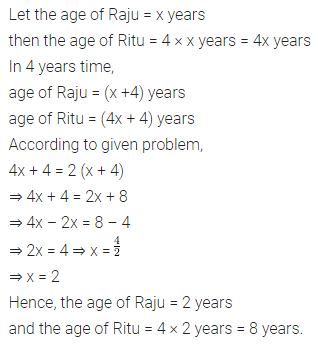Question 13.
A father is 7 times as old as his son. Two years ago, the father was 13 times as old as his son. How old are they now?
Solution: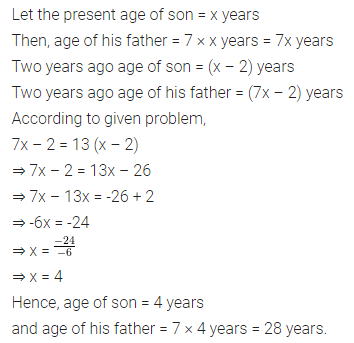Question 14.
The ages of Sona and Sonali are in the ratio 5 : 3. Five years hence, the ratio of their ages will be 10 : 7. Find their present ages.
Solution: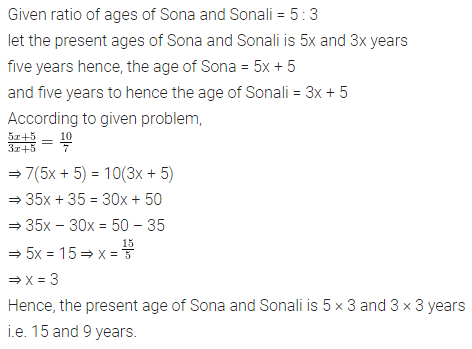Question 15.
An employee works in a company on a contract of 30 days on the condition that he will receive ₹200 for each day he works and he will be fined ₹20 for each day he is absent. If he receives ₹3800 in all, for how many days did he remain absent?
Solution: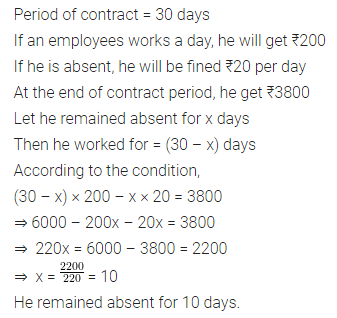Question 16.
I have a total of ₹300 in coins of denomination ₹1, ₹2 and ₹5. The number of coins is 3 times the number of ₹5 coins. The total number of coins is 160. How many coins of each denomination are with me?
Solution: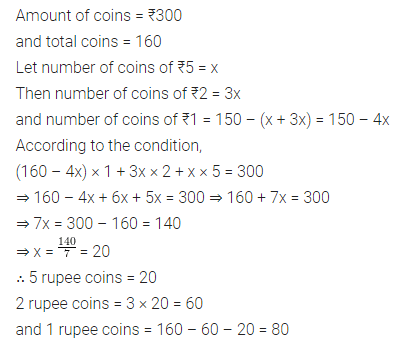Question 17.
A local bus is carrying 40 passengers, some with ₹5 tickets and the remaining with ₹7.50 tickets. If the total receipts from these passengers are ₹230, find the number of passengers with ₹5 tickets.
Solution:Question 18.
On a school picnic, a group of students agree to pay equally for the use of a full boat and pay ₹10 each. If there had been 3 more students in the group, each would have paid ₹2 less. How many students were there in the group?
Solution: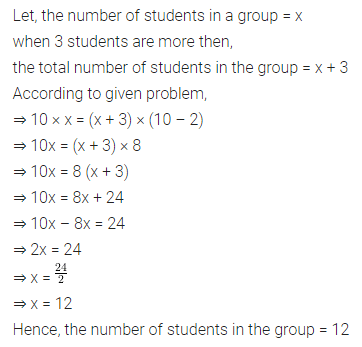Question 19.
Half of a herd of deer are grazing in the field and three-fourths of the remaining are playing nearby. The rest 9 are drinking water from the pond. Find the number of deer in the herd.
Solution:Question 20.
Sakshi takes some flowers in a basket and visits three temples one by one. At each temple, she offers one-half of the flowers from the basket. If she is left with 6 flowers at the end, find the number of flowers she had in the beginning.
Solution:Question 21.
Two supplementary angles differ by 50°. Find the measure of each angle.
Solution: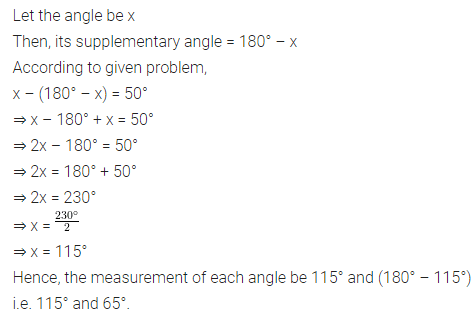Question 22.
If the angles of a triangle are in the ratio 5 : 6 : 7, find the angles.
Solution: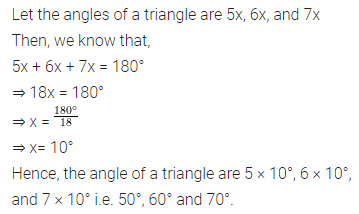Question 23.
Two equal sides of an isosceles triangle are 3x – 1 and 2x + 2 units. The third side is 2x units. Find x and the perimeter of the triangle.
Solution:Question 24.
If each side of a triangle is increased by 4 cm, the ratio of the perimeters of the new triangle and the given triangle is 7 : 5. Find the perimeter of the given triangle.
Solution: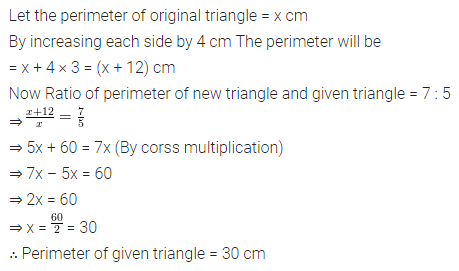Question 25.
The length of a rectangle is 5 cm less than twice its breadth. If the length is decreased by 3 cm and breadth increased by 2 cm, the perimeter of the resulting rectangle is 72 cm. Find the area of the original rectangle.
Solution: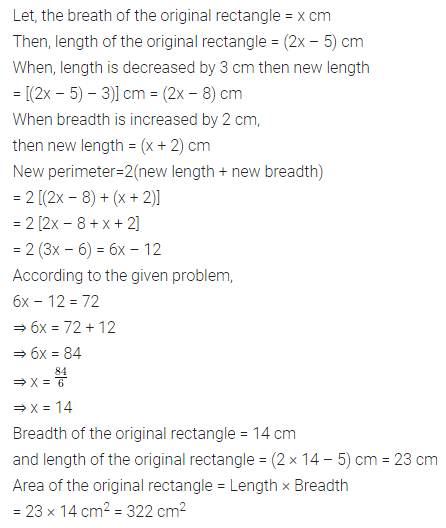Question 26.
A rectangle is 10 cm long and 8 cm wide. When each side of the rectangle is increased by x cm, its perimeter is doubled. Find the equation in x and hence find the area of the new rectangle.
Solution: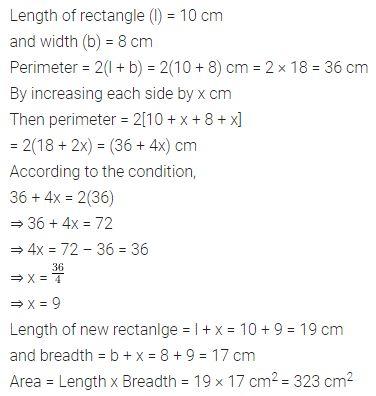Question 27.
A steamer travels 90 km downstream in the same time as it takes to travel 60 km upstream. If the speed of the stream is 5 km/hr, find the speed of the streamer in still water.
Solution:Question 28.
A steamer goes downstream and covers the distance between two ports in 5 hours while it covers the same distance upstream in 6 hours. If the speed of the stream is 1 km/h, find the speed of the streamer in still water and the distance between two ports.
Solution: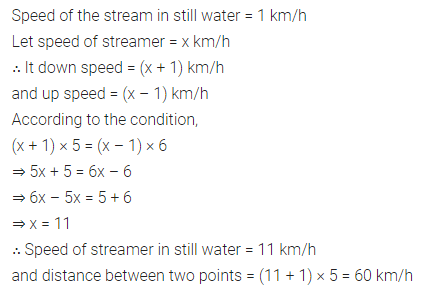Question 29.
Distance between two places A and B is 350 km. Two cars start simultaneously from A and B towards each other and the distance between them after 4 hours is 62 km. If the speed of one car is 8 km/h less than the speed of other cars, find the speed of each car.
Solution: